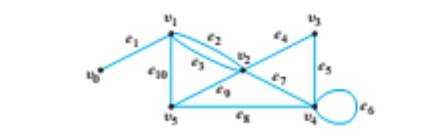Chapter 10.1, Problem 1ES### Discrete Mathematics With Applicat...

5th Edition
EPP + 1 other
ISBN: 9781337694193

#### Solutions

Chapter
Section### Discrete Mathematics With Applicat...

5th Edition
EPP + 1 other
ISBN: 9781337694193
Textbook Problem
8 views

# In the graph below, determine whether the following walks are trails, paths, closed walks, circuits, simple circuits, or just walks. v0e1v1e10v5e9v2e2v1 v4e7v2e9v5e10v1e3v2e9v5 v2 v5v2v3v4v5 v2v3v4v5v2v4v3v2 e2e8e10e3To determine

(a)

In the graph below, determine whether the following walks are trails, paths, closed walks, circuits, simple circuits, or just walks.

Explanation

Given information:

Calculation:

We know that:

A walk from v to w is a finite sequence of alternatively adjacent vertices and edges of a graph.

A trail from v to w is a walk that does not contain a repeated edge.

A path from v to w is a trial that does not contain a repeated vertex.

A closed walk is a walk that starts and ends at the same vertex.

A circuit is a closed walk with at least one edge and no repeated edges.

A simple circuit is a circuit with no repeated vertices except for the first and last vertex.

We have: v0e1v1e10v5e9v2e2v1.

The walk v0e1v1e10v5e9v2e2v1 is a trial, because we note that the walk does not contain a repeated edge (as the edges are e1,e10,e9 and e2).

The walk v0e1v1e10v5e9v2e2v1 is not a path, because we not that the walk contains the vertex v1 twice.

The walk v0e1v1e10v5e9v2e2v1 is not a closed walk, because the first vertex v0 is different from the last vertex v1

To determine

(b)

In the graph below, determine whether the following walks are trails, paths, closed walks, circuits, simple circuits, or just walks.

To determine

(c)

In the graph below, determine whether the following walks are trails, paths, closed walks, circuits, simple circuits, or just walks.

To determine

(d)

In the graph below, determine whether the following walks are trails, paths, closed walks, circuits, simple circuits, or just walks.

To determine

(e)

In the graph below, determine whether the following walks are trails, paths, closed walks, circuits, simple circuits, or just walks.

To determine

(f)

In the graph below, determine whether the following walks are trails, paths, closed walks, circuits, simple circuits, or just walks.

### Still sussing out bartleby?

Check out a sample textbook solution.

See a sample solution

#### The Solution to Your Study Problems

Bartleby provides explanations to thousands of textbook problems written by our experts, many with advanced degrees!

Get Started